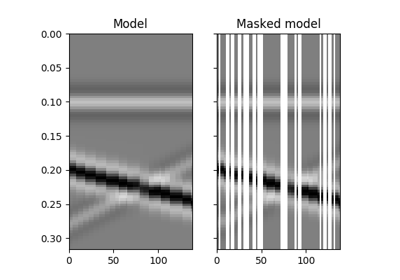# pylops.waveeqprocessing.SeismicInterpolation¶

pylops.waveeqprocessing.SeismicInterpolation(data, nrec, iava, iava1=None, kind='fk', nffts=None, sampling=None, spataxis=None, spat1axis=None, taxis=None, paxis=None, p1axis=None, centeredh=True, nwins=None, nwin=None, nover=None, design=False, engine='numba', dottest=False, **kwargs_solver)[source]

Seismic interpolation (or regularization).

Interpolate seismic data from irregular to regular spatial grid. Depending on the size of the input data, interpolation is either 2- or 3-dimensional. In case of 3-dimensional interpolation, data can be irregularly sampled in either one or both spatial directions.

Parameters: data : np.ndarray Irregularly sampled seismic data of size $$[n_{r_y} (\times n_{r_x} \times n_t)]$$ nrec : Number of elements in the regularly sampled (reconstructed) spatial array, $$n_{R_y}$$ for 2-dimensional data and $$(n_{R_y}, n_{R_x})$$ for 3-dimensional data iava : Integer (or floating) indices of locations of available samples in first dimension of regularly sampled spatial grid of interpolated signal. The pylops.basicoperators.Restriction operator is used in case of integer indices, while the pylops.signalprocessing.Iterp operator is used in case of floating indices. iava1 : list or numpy.ndarray, optional Integer (or floating) indices of locations of available samples in second dimension of regularly sampled spatial grid of interpolated signal. Can be used only in case of 3-dimensional data. kind : str, optional Type of inversion: fk (default), spatial, radon-linear, radon-parabolic or , radon-hyperbolic and sliding nffts : int or tuple, optional nffts : tuple, optional Number of samples in Fourier Transform for each direction. Required if kind='fk' sampling : tuple, optional Sampling steps dy (, dx) and dt. Required if kind='fk' or kind='radon-lin' spataxis : np.ndarray, optional First spatial axis. Required for kind='radon-lin', can also be provided instead of sampling for kind='fk' spat1axis : np.ndarray, optional Second spatial axis. Required for kind='radon-lin', can also be provided instead of sampling for kind='fk' timeaxis : np.ndarray, optional Time axis. Required for kind='radon-lin', can also be provided instead of sampling for kind='fk' paxis : np.ndarray, optional First Radon axis. Required for kind='radon-linear', kind='radon-parabolic', kind='radon-hyperbolic' and kind='sliding' p1axis : np.ndarray, optional Second Radon axis. Required for kind='radon-linear', kind='radon-parabolic' and kind='radon-hyperbolic' and kind='sliding' centeredh : bool, optional Assume centered spatial axis (True) or not (False). Required for kind='radon-linear', kind='radon-parabolic' and kind='radon-hyperbolic' nwins : int or tuple, optional Number of windows. Required for kind='sliding' nwin : int or tuple, optional Number of samples of window. Required for kind='sliding' nover : int or tuple, optional Number of samples of overlapping part of window. Required for kind='sliding' design : bool, optional Print number of sliding window (True) or not (False) when using kind='sliding' engine : str, optional Engine used for Radon computations (numpy or numba) dottest : bool, optional Apply dot-test **kwargs_solver Arbitrary keyword arguments for pylops.optimization.leastsquares.RegularizedInversion solver if kind='spatial' or pylops.optimization.sparsity.FISTA solver otherwise recdata : np.ndarray Reconstructed data of size $$[n_{R_y} (\times n_{R_x} \times n_t)]$$ recprec : np.ndarray Reconstructed data in the sparse or preconditioned domain in case of kind='fk', kind='radon-linear', kind='radon-parabolic', kind='radon-hyperbolic' and kind='sliding' cost : np.ndarray Cost function norm KeyError If kind is neither spatial, fl, radon-linear, radon-parabolic, radon-hyperbolic nor sliding

Notes

The problem of seismic data interpolation (or regularization) can be formally written as

$\mathbf{y} = \mathbf{R} \mathbf{x}$

where a restriction or interpolation operator is applied along the spatial direction(s). Here $$\mathbf{y} = [\mathbf{y}_{R1}^T, \mathbf{y}_{R2}^T,..., \mathbf{y}_{RN^T}]^T$$ where each vector $$\mathbf{y}_{Ri}$$ contains all time samples recorded in the seismic data at the specific receiver $$R_i$$. Similarly, $$\mathbf{x} = [\mathbf{x}_{r1}^T, \mathbf{x}_{r2}^T,..., \mathbf{x}_{rM}^T]$$, contains all traces at the regularly and finely sampled receiver locations $$r_i$$.

Several alternative approaches can be taken to solve such a problem. They mostly differ in the choice of the regularization (or preconditining) used to mitigate the ill-posedness of the problem:

• spatial: least-squares inversion in the original time-space domain with an additional spatial smoothing regularization term, corresponding to the cost function $$J = ||\mathbf{y} - \mathbf{R} \mathbf{x}||_2 + \epsilon_\nabla \nabla ||\mathbf{x}||_2$$ where $$\nabla$$ is a second order space derivative implemented via pylops.basicoperators.SecondDerivative in 2-dimensional case and pylops.basicoperators.Laplacian in 3-dimensional case
• fk: L1 inversion in frequency-wavenumber preconditioned domain corresponding to the cost function $$J = ||\mathbf{y} - \mathbf{R} \mathbf{F} \mathbf{x}||_2$$ where $$\mathbf{F}$$ is frequency-wavenumber transform implemented via pylops.signalprocessing.FFT2D in 2-dimensional case and pylops.signalprocessing.FFTND in 3-dimensional case
• radon-linear: L1 inversion in linear Radon preconditioned domain using the same cost function as fk but with $$\mathbf{F}$$ being a Radon transform implemented via pylops.signalprocessing.Radon2D in 2-dimensional case and pylops.signalprocessing.Radon3D in 3-dimensional case
• radon-parabolic: L1 inversion in parabolic Radon preconditioned domain
• radon-hyperbolic: L1 inversion in hyperbolic Radon preconditioned domain
• sliding: L1 inversion in sliding-linear Radon preconditioned domain using the same cost function as fk but with $$\mathbf{F}$$ being a sliding Radon transform implemented via pylops.signalprocessing.Sliding2D in 2-dimensional case and pylops.signalprocessing.Sliding3D in 3-dimensional case

## Examples using pylops.waveeqprocessing.SeismicInterpolation¶11. Seismic regularization